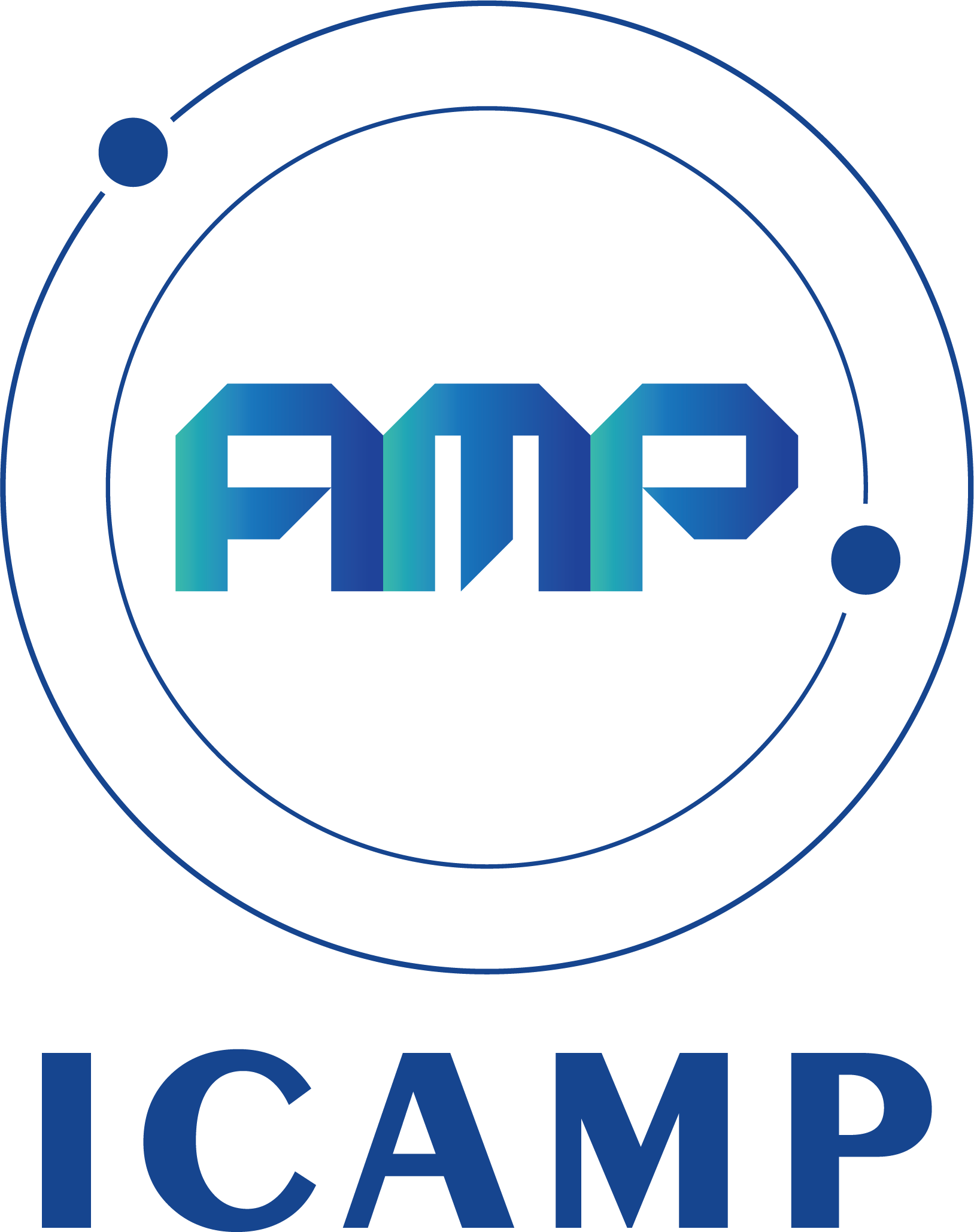2023 2nd International Conference on Applied Mathematics and Physics (ICAMP 2023)
Call For Papers
Home / Call For Papers

2023 2nd International Conference on Applied Mathematics and Physics (ICAMP 2023) will bring together leading researchers, engineers and scientists in the domain of interest from around the world.

Topics of interest for submission include, but are not limited to:

01. Applied Mathematics

• Riemannian surfaces and Riemannian geometry

• Combinatorial mathematics

• Finite group

• Geometry and global differential geometry

• Elementary algebraic topology

• Cryptography

• Differential dynamical system

• Harmonic analysis selected lecture

• Statistical Software (SAS)

• Nonparametric statistics

• Robust statistical analysis

• Experimental design and quality control

• Mathematical model

• Topology

• Differential geometry

• Partial differential equation

• Simulation and Monte-Carlo methods

• Mathematical analysis

• Function theory

• Discrete mathematics

• Numerical methods and computer technology

• Mathematics experiment

• Differential manifold

• Lie group and representation

• Linear algebra

• Matrix theory

• Vector analysis

• Complex analysis

• Differential equation

• Laplace transform

• Fourier analysis

02. Physics

• Physics and applied Physics

• Nuclear physics

• Acoustic

• Systems Science and Engineering

• Quantum information science

• Mechanics and theoretical mechanics

• Optical

• Electromagnetism

• Atomic physics

• Electrodynamics

• Quantum mechanics

• Solid state physics

• Structure and physical properties

• Electrical and electronic technology

• Semiconductor physics

• Optoelectronics

• Experimental methods of nuclear physics

• Nuclear electronics

• Engineering noise control

• Electroacoustic technology

• Sound technology

• Circuit principle and analysis

• Electronic circuits and experiments

• Signal analysis and processing

• Quantum electronic informatics

• Optical quantum informatics

• Space Science and Technology